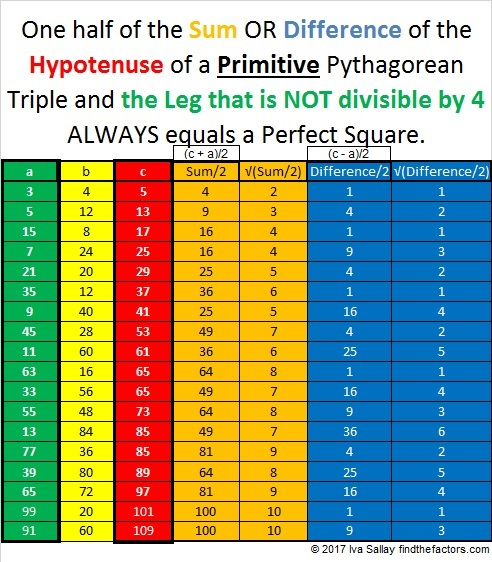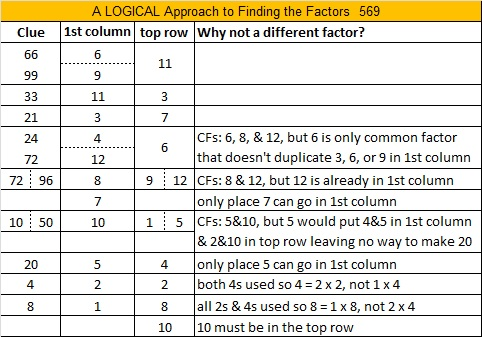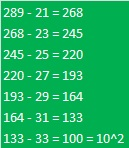# 857 Why do Primitive Pythagorean Triples Do That?

29² + 4² = 857, so 857 is the hypotenuse of a Pythagorean triple that just happens to be primitive:

• 232-825-857 which was calculated from 2(29)(4), 29² – 4², 29² + 4².

Now, one of the legs of a primitive Pythagorean triple will be divisible by 4. In this case that number is 232. As I indicated when I wrote 821 Why Do Primitive Pythagorean Triples Do That? The sum of the number divisible by 4 in a primitive Pythagorean triple and the hypotenuse always equals a perfect square. So 232 + 857 = 1089 = 33².

This week I noticed something about the OTHER leg that might be even more amazing! The other leg in the triple, 825, is not divisible by 4, but get this: One half of the sum of a primitive’s hypotenuse and that leg that is NOT divisible by 4 also equals a square number: For example, (857 + 825)/2 = 1681/2 = 841 = 29².

But not only that, one half of the DIFFERENCE of a primitive’s hypotenuse and that leg that is NOT divisible by 4 equals a perfect square, too: (857 – 825)/2 = 32/2 = 16 = 4².

Now 29² and 4² look a little familiar to me today. Hmm… We’ve seen those two numbers together earlier in this post!

It seems that for Primitive Pythagorean Triples, what goes around comes around!

Check out the sums and difference of these other primitive Pythagorean triples:Go ahead, try it with any other primitive triple on this list or otherwise!

If we let s = √(Sum/2) and let d = √(Difference/2), then we can rewrite some Pythagorean triple like this:

• s² – d², 2sd, s² + d², so s² and d² are REALLY significant perfect squares!

Besides being the hypotenuse of a primitive Pythagorean triple, here are some other facts about the number 857:

857 is the sum of three consecutive prime numbers: 281 + 283 + 293.

857 is also the sum of the nineteen prime numbers from 11 to 83.

• 857 is a prime number.
• Prime factorization: 857 is prime.
• The exponent of prime number 857 is 1. Adding 1 to that exponent we get (1 + 1) = 2. Therefore 857 has exactly 2 factors.
• Factors of 857: 1, 857
• Factor pairs: 857 = 1 × 857
• 857 has no square factors that allow its square root to be simplified. √857 ≈ 29.27456

How do we know that 857 is a prime number? If 857 were not a prime number, then it would be divisible by at least one prime number less than or equal to √857 ≈ 29.3. Since 857 cannot be divided evenly by 2, 3, 5, 7, 11, 13, 17, 19, 23, or 29, we know that 857 is a prime number.

Here’s another way we know that 857 is a prime number: Since its last two digits divided by 4 leave a remainder of 1, and 29² + 4² = 857 with 29 and 4 having no common prime factors, 857 will be prime unless it is divisible by a prime number Pythagorean triple hypotenuse less than or equal to √857 ≈ 29.3. Since 853 is not divisible by 5, 13, 17, or 29, we know that 857 is a prime number.

# 569 and Level 5

When we divide the last two digits of prime number 569 by 4, we get a remainder of one. That means that 569 is the sum of two square numbers, specifically, 20² + 13² = 569.

From those two square numbers we will obtain 569 as the hypotenuse of the primitive Pythagorean triple 231-520-569:

• 20² – 13² = 231
• 2 x 13 x 20 = 520
• 20² + 13² = 569Print the puzzles or type the solution on this excel file: 12 Factors 2015-07-27

—————————————————————————————————

• 569 is a prime number.
• Prime factorization: 569 is prime.
• The exponent of prime number 569 is 1. Adding 1 to that exponent we get (1 + 1) = 2. Therefore 569 has exactly 2 factors.
• Factors of 569: 1, 569
• Factor pairs: 569 = 1 x 569
• 569 has no square factors that allow its square root to be simplified. √569 ≈ 23.85372How do we know that 569 is a prime number? If 569 were not a prime number, then it would be divisible by at least one prime number less than or equal to √569 ≈ 23.8. Since 569 cannot be divided evenly by 2, 3, 5, 7, 11, 13, 17, 19, or 23, we know that 569 is a prime number.# 389 How to Know if a Prime Number is the Hypotenuse in a Primitive Pythagorean Triple

• 389 is a prime number.
• Prime factorization: 389 is prime.
• The exponent of prime number 389 is 1. Adding 1 to that exponent we get (1 + 1) = 2. Therefore 389 has exactly 2 factors.
• Factors of 389: 1, 389
• Factor pairs: 389 = 1 x 389
• 389 has no square factors that allow its square root to be simplified. √389 ≈ 19.723How do we know that 389 is a prime number? If 389 were not a prime number, then it would be divisible by at least one prime number less than or equal to √389 ≈ 19.723. Since 389 cannot be divided evenly by 2, 3, 5, 7, 11, 13, 17, or 19, we know that 389 is a prime number.

If a Pythagorean triple is a primitive, then its hypotenuse is always an odd number. Sometimes it is also a prime number. How can we know if a particular prime number, such as 389, is ever the hypotenuse of a Pythagorean triple?

Divide the last two digits of the prime number by 4 and look at the remainder:

• If the remainder is 3, then that prime number will not ever be the hypotenuse of any Pythagorean triple.
• If the remainder is 1, then it is the hypotenuse of a primitive Pythagorean triple! Find the proof here.

The last two digits of 389 is 89, and 89 ÷ 4 = 22 R1, so 389 and every other prime number ending in 89 is the hypotenuse of a primitive Pythagorean triple. What are the other two sides of this particular triangle? First we will figure out what two square numbers equal 389, then we will use those two numbers to figure out what two square numbers equal 389 squared:

Have you ever noticed that 1 is the first square number, 1 + 3 or 4 is also a square number, and 1 + 3 + 5 or 9 is yet another square number? This principle can be summarized by writing 1 + 3 + 5 + . . . + (2n – 1) = n squared.

I’ll use this fact to determine when 389 is the hypotenuse of a primitive Pythagorean triple. I’ll subtract 1 from 389, then 3 from that result, and continue to subtract the next odd number until I get a perfect square. I should find the perfect square before I reach 194 (about half of 389).

So let’s begin:At this point I know that 17² + another square equals 389. There are a few methods I can use to find the other perfect square.

• I can find the other square by subtracting: 389 – 17²
• I can take the last odd number used, 19, add one, then divide by two and then square that number.
• Or I can continue subtracting odd numbers as before:All of these methods give us 100 or 10² as the second perfect square. Notice that (33 + 1)/2 = 17 as well.

Now we have enough information to determine the other two numbers that make up the Pythagorean triple with 389 as the hypotenuse.

• All of the numbers, a – b – c, in the triple can be found using the formulas Euclid provided more than 2000 years ago. Let 10 = n; and 17 = m.
• a = m² – n² = 17² – 10² = 189
• b = 2mn = 2(10)(17) = 340
• c = m² + n² = 17² + 10² = 389
• thus 189 – 340 – 389 make a primitive Pythagorean triple. We know it is a primitive because 389 is prime and has no other factors besides one and itself.

# 383 is in only one Pythagorean triple, and it’s primitiveA Primitive Threesome https://www.mycomicshop.com/search?TID=243401

Although it would take awhile to count to 383, it is still a relatively small number. If you scroll down, you will see that it is also a prime number. Since all odd numbers greater than one are the short legs of a primitive Pythagorean triple, 383 is also. I will explain how to find that primitive triple using the Flintstones, the primitive yet modern stone age family as an analogy. You can use this method on ANY odd number greater than one to find a primitive triple:

• 383 is like Pebbles the shortest one in the family
• If we round down (383^2)/2 or 73344.5 to 73,344, we get a much bigger number, much like Fred is much taller than Pebbles in the family
• If we round the same expression up, we get 73,345, much like Wilma is just a little taller than Fred.
• 383 – 73344 – 73345 is a primitive Pythagorean triple just as Pebbles, Fred, and Wilma are a primitive yet modern stone age family of three. Test it out: (383^2) + (73344^2) = (73345^2)

Prime numbers can be one of the legs of only one Pythagorean triple, and it will always be a primitive. Sometimes prime numbers are the hypotenuse of a primitive Pythagorean triple, but 383 isn’t. In a future post, I’ll explain how to determine if a prime number is ever the hypotenuse.

Here is 383’s factoring information:

• 383 is a prime number.
• Prime factorization: 383 is prime.
• The exponent of prime number 383 is 1. Adding 1 to that exponent we get (1 + 1) = 2. Therefore 383 has exactly 2 factors.
• Factors of 383: 1, 383
• Factor pairs: 383 = 1 x 383
• 383 has no square factors that allow its square root to be simplified. √383 ≈ 19.570How do we know that 383 is a prime number? If 383 were not a prime number, then it would be divisible by at least one prime number less than or equal to √383 ≈ 19.570. Since 383 cannot be divided evenly by 2, 3, 5, 7, 11, 13, 17, or 19, we know that 383 is a prime number.